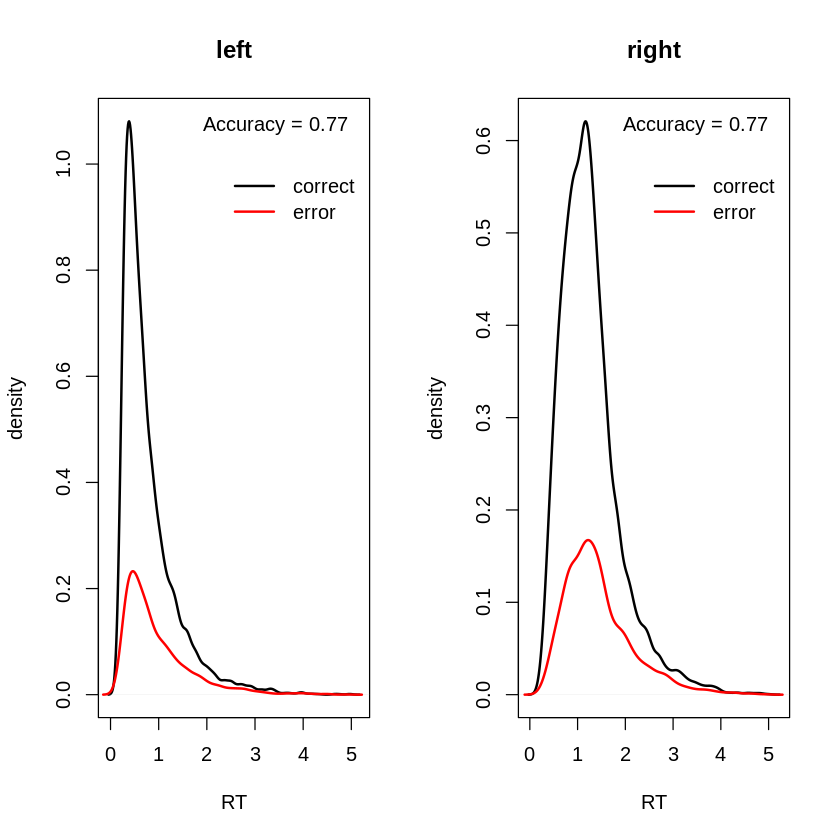# RunDMC in a jupyter notebook

Sorry, it's not about how to invite runDMC rappers to feature your notebook, rather it is about using the package "dynamic models of choice" (runDMC), to fit decision making models to your behavioral (and physiological) data within the environment of Jupyter.

Why you ask ? Well, I'm allergic to development environment such as Rstudio or Rmarkdown as they are, to me, not practical enough when it comes to explore your data or your analysis pipeline while keeping a record of everything. For that I prefer the jupyter environment but installing R and it's packages can be tricky, especially with complex architectures such as the one required by runDMC. Therefore I wrote this short tutorial in case others want to use the ability of jupyter but still rely on runDMC to do your decision data anaysis.

# Setup¶

First we will ensure that packages from conda are downloaded from the conda-forge repositories that has a larger collection of packages than default conda repositories. For that, in a terminal (for linux and Mac users) or using the anaconda prompt (for windows) launch the following command :

conda config --add channels conda-forge
conda config --set channel_priority strict



Then we will simultaneously create a new environment called "DMC" with the available and required packages from conda-forge :

conda create -n DMC r-loo r-hypergeo r-statmod r-pracma r-snowfall r-numDeriv r-vioplot r-ggplot2 r-gridExtra r-truncdist r-msm r-rlecuyer r-laplacesdemon r-mvtnorm r-matrix jupyter #That will take a while



However a few of the required packages are not contained in the conda forge rep (rtdists, stringr, Brobdingnag) . We therefore have to launch an R session in the DMC environment to install them afterwards. For that first launch an R session by previously activating our new conda env :

conda activate DMC
R
install.packages("Brobdingnag") # This will prompt a screen requiring to select a server from which you will download, just select the nearest one
install.packages("stringr")
install.packages("rtdists")



Then we will install a modified version of the coda package that allows for plotting of priors with plot.dmc. For that download the zip file from the latest DMC version, extract it in a relevant path and then move to that working directory within the R session, in my case and for the version 190819 it goes as follows :

setwd("~/DMC_190819")



And install the coda package :

install.packages("packages/coda_0.19-3.tar.gz",repos=NULL,type="source")



Then R and jupyter packages are not interfacing very well you need a last step to provide jupyter with the relevant locations for the R packages :

 install.packages('IRkernel')
IRkernel::installspec()



Et voilà ! You're ready to go and use runDMC and the associated tutorials :)

Let me know in case you run into troubles.

See below (or download it here) for an example of the notebook taken from DMC tutorial dmc_1_5_ddm.R :

# Demo : DMC Lesson 1 Models and Data¶

Note: Before running these scripts, the current working directory must be set to the top-level folder containing the dmc and tutorial subfolders

## Lesson 1.5 Exploring the DDM model¶

This lesson is about familiarising yourself with the DDM model and its parameters, and how you can create different scenarios with different parameter values. See Ratcliff, R., & McKoon, G. (2008). The diffusion decision model: Theory and data for two-choice decision tasks. Neural Computation, 20(4), 873–922.

In [ ]:
rm(list=ls()); par(mfrow = c(1,1))
source ("dmc/dmc.R")



Start with a simple design with a binary stimulus factor, S (e.g, left vs. right motion) and corresponding responses

In :
factors=list(S=c("left","right"))
responses=c("LEFT","RIGHT")
match.map=list(M=list(left="LEFT",right="RIGHT"))


NB1: See ?ddiffusion for rtdists help on parameters. However note that we differ from rtdists in defining z (the start point) relative to a (the boundary). That is, if the absolute start point is Z then z = Z/a, where 0 < z < 1. Similarly, sz, start point noise (i.e., the width of the uniform distribution around z of start points) is defined as a proportion of a, so if SZ is the absolute start point distribution width then sz = SZ/a. Note also that t0 is the LOWER BOUND of the non-decision time distribution (in this case this is true in both rtdists and in DMC) so, that mean non-decision time is t0 + st/2, where st is the width of the uniform distribution of non-decision time. These parameterization of z, sz and t0 are all adopted so that the parameters can be restricted to their proper range by priors bounded by absolute values (i.e, 0 < z < 1, 0 < sz < 1, t0 > 0 and st > 0) rather than having to provide relative bounds (e.g., Z < a etc.)

NB2: Like the LNR, for the DDM there is no need to fix a parameter (by default moment-to-moment variability s=1 is assumed, see rtdists). Constants are set here just to focus on the computationally faster version with no non-decision variability (st0=0) and with no difference in non-decision time between responses (d=0). When st0>0 it gets slow!

NB3: Because there is only one accumulator the M and R factors cannot appear in p.map

NB4: Negative v is mapped to the error boundary and positive to correct boundary, so estimates are generally positive.

NB5: Note that the left response corresponds to the bottom boundary (at 0) and the right response to the top boundary (at a)

NB6: The underlying code calculating the DDM likelihood uses numerical integration in C and can crash when given parameters outside and even sometimes very near the boundaries over which each parameter is defined.

 In order to avoid these problems parameters are checked as follows and
a zero likelihood returned (see transfrom.dmc in ddm.R)
{
(p$a<0) | (p$z <1e-6) | (p$z >.999999) | (p$t0<1e-6)  |
(p$sz<0) | (p$st0<0)    | (p$sv<0) | (p$sz>1.999999*min(c(p$z,1-p$z)))
}

Note that sz has a rather complicated from becasue we have to make sure
that both 0 < Z - SZ/2 and Z + SZ/2 < a. This is the same as 0 < z-sz/2
and z+sz/2 < 1 or jointly sz/2 < min(z,1-z).



NB7: The d parameter (difference in t0 between responses, positive values = faster for upper threshold, negative = faster for lower threshold) from rtdists is re-defined in a relative sense i.e., d_rtdists = t0 * d_dmc so it is easy to make sure that non-decison time for both responses is always positive by bounding -2 < d_dmc < 2.

### Simple no factor effects model¶

In :
model <- model.dmc(constants=c(st0=0,d=0),type="rd",
p.map=list(a="1",v="1",z="1",d="1",
sz="1",sv="1",t0="1",st0="1"),
match.map=list(M=list(left="LEFT",right="RIGHT")),
factors=list(S=c("left","right")),
responses=c("LEFT","RIGHT"))

Parameter vector names are: ( see attr(,"p.vector") )
 "a"  "v"  "z"  "sz" "sv" "t0"

Constants are (see attr(,"constants") ):
st0   d
0   0

Model type = rd



#### 1) In this example accuracy is a bit above 75%¶

In :
p.vector  <- c(a=2,v=1,z=0.5,sv=1,sz=0.2,t0=.2)
data.model <- data.model.dmc(simulate.dmc(p.vector,model,n=1e4),model)
plot.score.dmc (data.model)

 0.77
FALSE  TRUE
1.07  0.88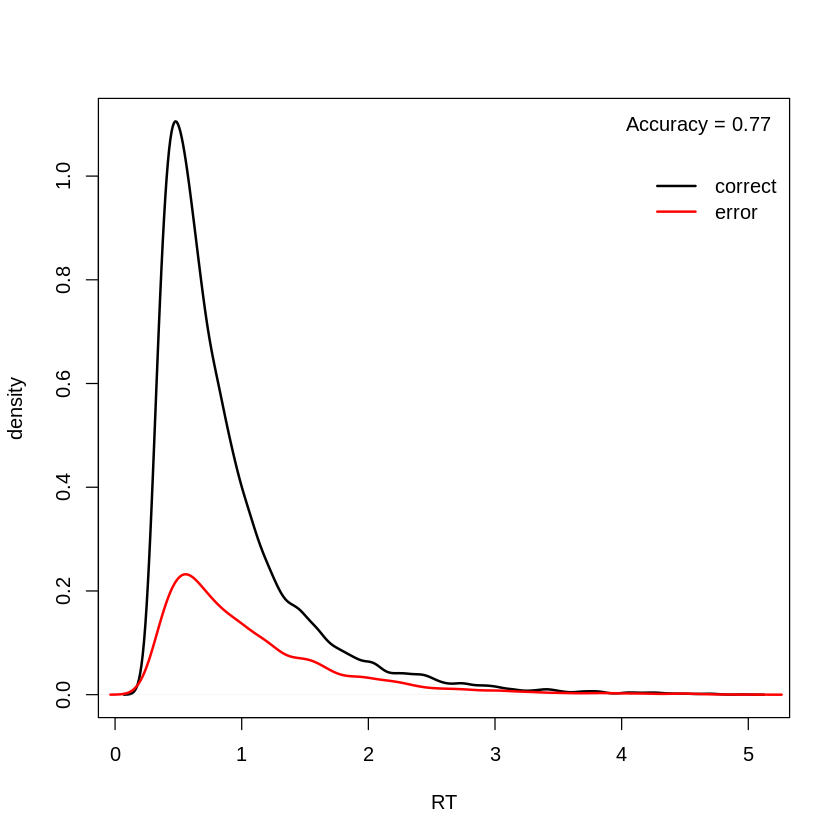#### Decreasing the threshold (a) decreases accuracy but increases speed¶

In :
p.vector  <- c(a=1,v=1,z=0.5,sv=1,sz=0.2,t0=.2)
data.model <- data.model.dmc(simulate.dmc(p.vector,model,n=1e4),model)
plot.score.dmc (data.model)

 0.69
FALSE  TRUE
0.43  0.41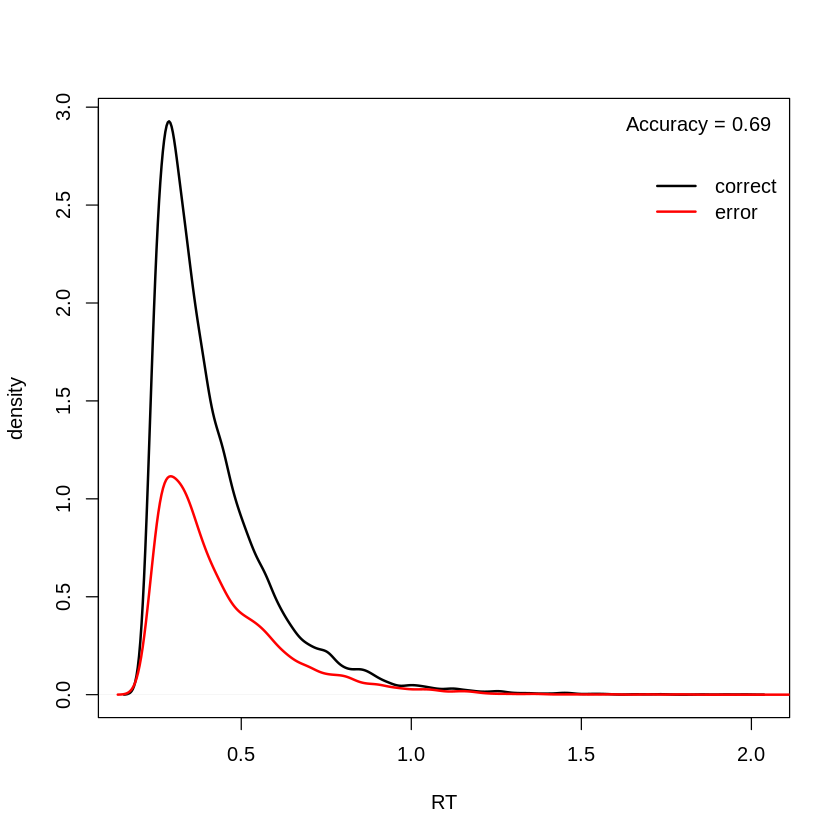#### 3) Increasing start-point noise (sz) also decreases accuracy and increases speed (NOTE: sz must always be < a/2, otherwise an error occurs)¶

In :
p.vector  <- c(a=2,v=1,z=0.5,sv=1,sz=.9,t0=.2)
data.model <- data.model.dmc(simulate.dmc(p.vector,model,n=1e4),model)
plot.score.dmc (data.model)

 0.72
FALSE  TRUE
0.75  0.76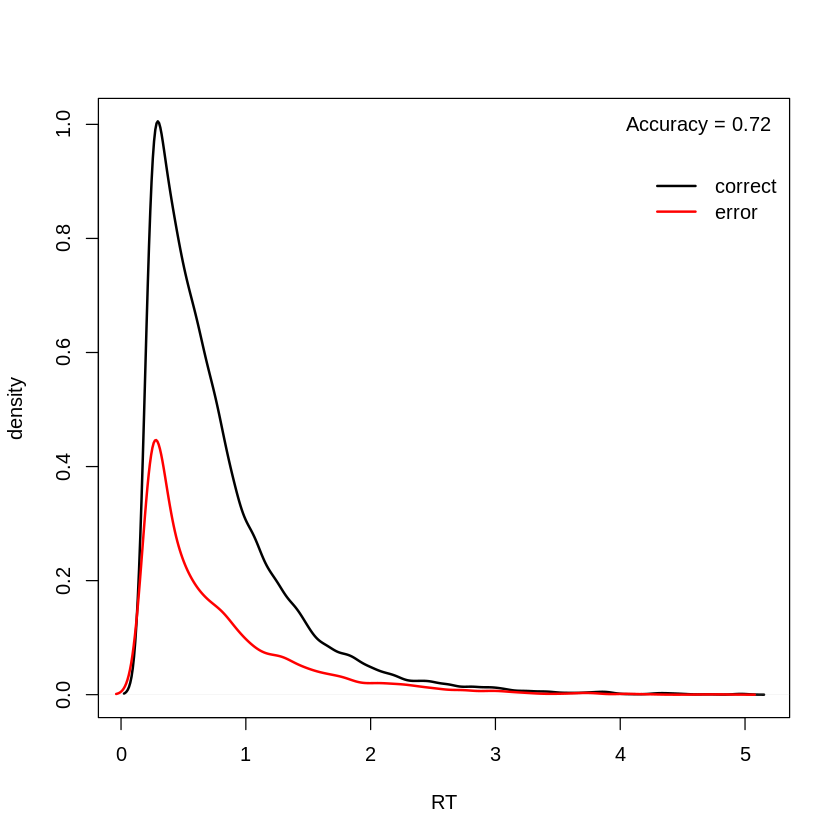#### 4) Decreasing v decreases accuracy and speed (mainly for correct responses in this case)¶

In :
p.vector  <- c(a=2,v=0.5,z=0.5,sv=1,sz=0.2,t0=.2)
data.model <- data.model.dmc(simulate.dmc(p.vector,model,n=1e4),model)
plot.score.dmc (data.model)

 0.65
FALSE  TRUE
1.06  0.95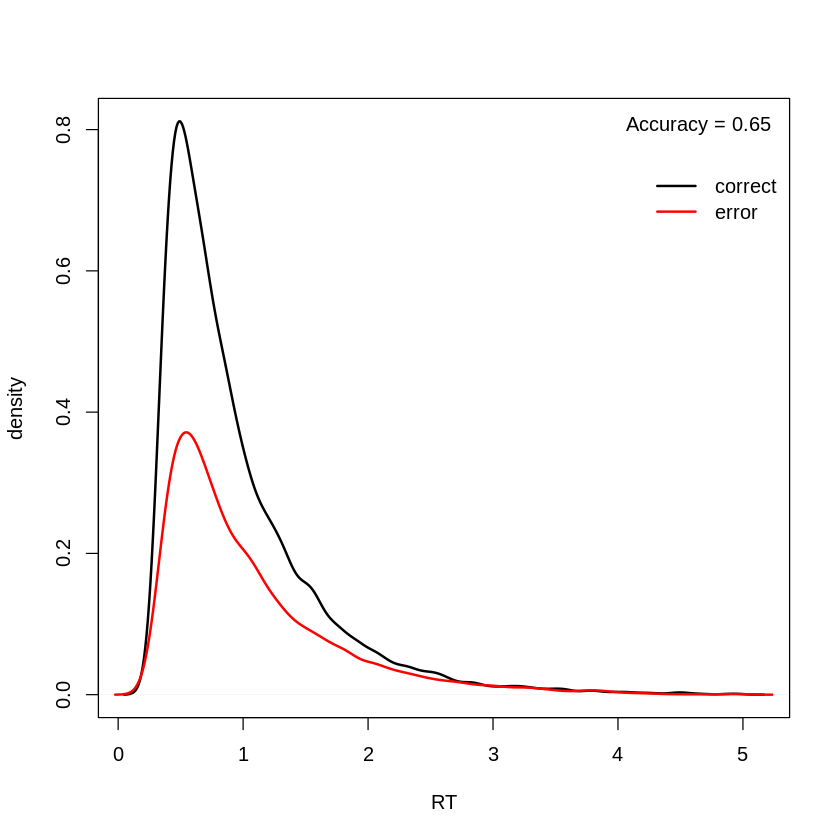#### 5) Increasing sv mainly decreases accuracy (also a little RT)¶

In :
p.vector  <- c(a=2,v=1,z=0.5,sv=1.5,sz=0.2,t0=.2)
data.model <- data.model.dmc(simulate.dmc(p.vector,model,n=1e4),model)
plot.score.dmc (data.model)

 0.71
FALSE  TRUE
0.98  0.80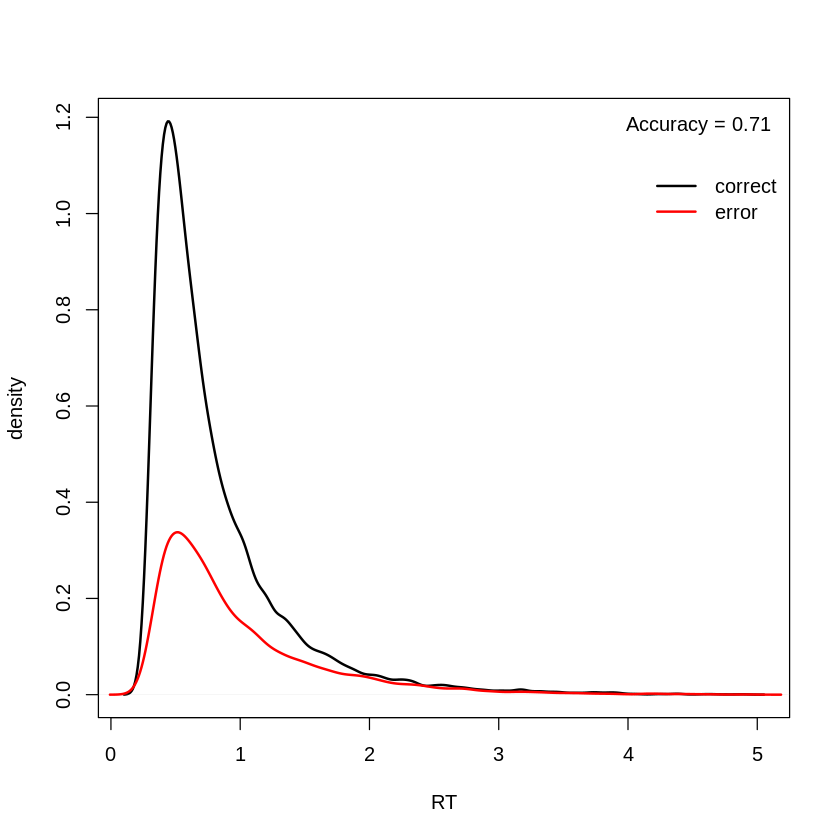#### 6) Increasing t0 decreases speed but has no effect on accuracy¶

In :
p.vector  <- c(a=2,v=1,z=0.5,sv=1,sz=0.2,t0=.4)
data.model <- data.model.dmc(simulate.dmc(p.vector,model,n=1e4),model)
plot.score.dmc (data.model)

 0.77
FALSE  TRUE
1.27  1.07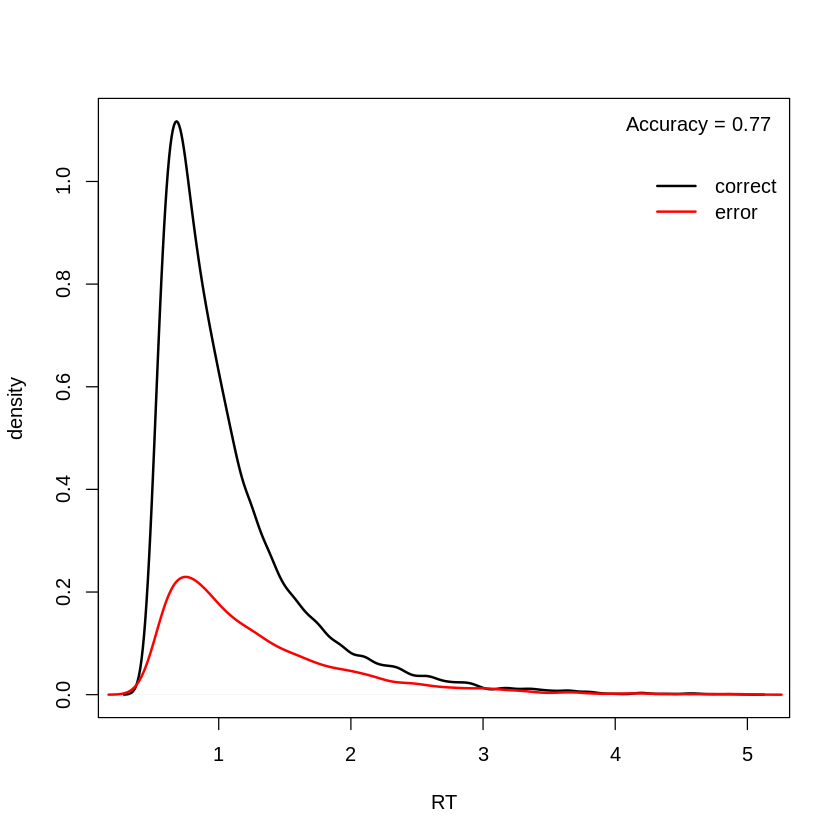Now lets look at the effects of non-decision noise, so st0 is no longer a constant. Just as in LBA t0 is the lower bound of non-decision time and t0+st0 is the upper bound (so mean non-decision time is t0 + st0/2).

In :
model <- model.dmc(constants=c(d=0),type="rd",
p.map=list(a="1",v="1",z="1",d="1",
sz="1",sv="1",t0="1",st0="S"),
match.map=list(M=list(left="LEFT",right="RIGHT")),
factors=list(S=c("left","right")),
responses=c("LEFT","RIGHT"))

Parameter vector names are: ( see attr(,"p.vector") )
 "a"         "v"         "z"         "sz"        "sv"        "t0"
 "st0.left"  "st0.right"

Constants are (see attr(,"constants") ):
d
0

Model type = rd



Accuracy is unaffected by variability increases.

In :
p.vector  <- c(a=2,v=1,z=0.5,sv=1,sz=0.2,t0=0.1,st0.left=0,st0.right=1)
data.model <- data.model.dmc(simulate.dmc(p.vector,model,n=1e4),model)


Score & plot

In :
correct <- data.model$S==tolower(data.model$R)


No effect on accuracy

In :
round(tapply(correct,data.model$S,mean),2)  left 0.77 right 0.77 Mean RT is increased by st0/2 In : round(tapply(data.model$RT,data.model$S,mean),2)  left 0.83 right 1.31 Variability for left stimuli less than for right In : round(tapply(data.model$RT,data.model$S,IQR),2) par(mfrow=c(1,2)) plot.cell.density(data.model[data.model$S=="left",],C=correct[data.model$S=="left"], xlim=c(0,5),main="left") plot.cell.density(data.model[data.model$S=="right",],C=correct[data.model\$S=="right"],
xlim=c(0,5),main="right")

left
0.62
right
0.72